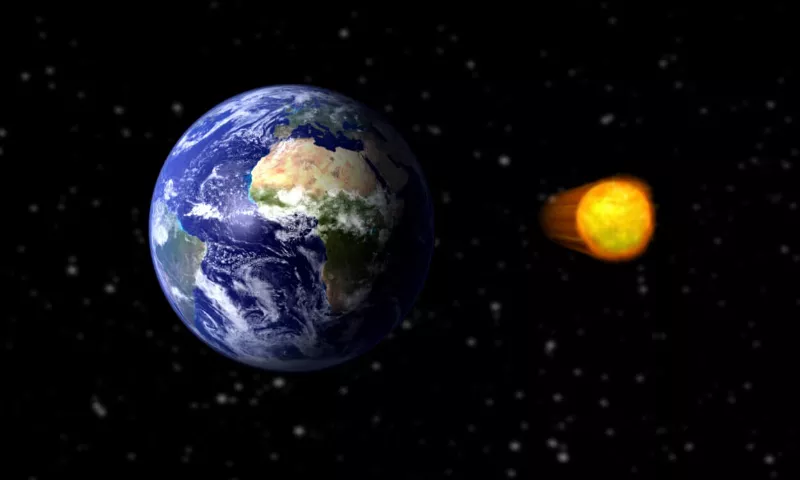### Earth's Velocity

The Earth's average velocity orbiting the sun is 110,000 km per hour.Share

Earth's average orbital speed is about 30 kilometers per second. In other units, that's about 19 miles per second, or 67,000 miles per hour, or 110,000 kilometers per hour (110 million meters per hour).

Let's calculate that. First of all, we know that in general, the distance you travel equals the speed at which you travel multiplied by the time (duration) of travel. If we reverse that, we get that the average speed is equal to the distance traveled over the time taken.

We also know that the time it takes for the Earth to go once around the Sun is one year. So, in order to know the speed, we just have to figure out the distance traveled by the Earth when it goes once around the Sun. To do that we will assume that the orbit of the Earth is circular (which is not exactly right, it is more like an ellipse, but for our purpose, a circle is close enough). So the distance traveled in one year is just the circumference of the circle.The cosmos contains approximately 100 - 200 billion galaxies.Humans can only see about 4% of the matter in the Universe.The only two planets in our solar system that do not have moons are Mercury and Venus.The sun orbits around the Milky Way at a speed of about 220 km (140 miles) per second.